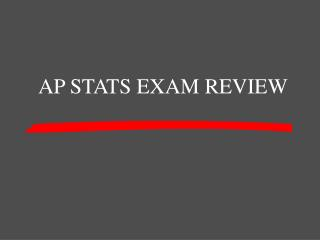DownloadDownload PresentationAP STATS EXAM REVIEW

AP STATS EXAM REVIEW

Télécharger la présentationAP STATS EXAM REVIEW

- - - - - - - - - - - - - - - - - - - - - - - - - - - E N D - - - - - - - - - - - - - - - - - - - - - - - - - - -
Presentation Transcript

1. AP STATS EXAM REVIEW

2. Chapter 2 Chapter 1 Chapter 3 and Chapter 4 Chapter 5 Chapter 6 100 100 100 100 100 200 200 200 200 200 300 300 300 300 300 400 400 400 400 400 500 500 500 500 500

3. These are the three terms you should always discuss when describing a set of data Categories

5. Skewed right Categories

6. Chapter 1 400 Points This is the formula for finding outliers in a set of data Categories

7. Chapter 1 400 Points Q1 -1.5IQR and Q3 + 1.5IQR Categories

8. Chapter 1 500 Points the formula for this measurement that is used to describe a set of data is to subtract the mean from each of the numbers in the data set, square that difference, find the sum of the differences, and then take the square root of the sums Categories

9. Chapter 1 500 Points Standard deviation Categories

10. Chapter 2 100 Points This is what the 95 means in the 68-95-99.7 rule Categories

11. Chapter 2 100 Points 95% of the data is within 2 standard deviations Categories

12. Chapter 2 200 Points this is the formula for the z score of a mean Categories

13. Chapter 2 200 Points (x-bar – mean)/standard devation Categories

14. Chapter 2 300 Points This is the area to the left of a z-score of 2.34 Categories

15. Chapter 2 300 Points Categories

16. Chapter 2 400 Points Probability for a two sided Z-score is 13.1%, this is the z-score Categories

17. Chapter 2 400 Points 1.52 Categories

18. Chapter 2 500 Points In 2000, the scores of men on the math SAT followed a normal distribution with mean 533 and standard deviation 115. This is the percentage of men who scored 750 or better Categories

19. Chapter 2 500 Points 2.94% Categories

20. Chapter 3/4 100 Points This is the equation for the LSRL Categories

21. Chapter ¾ 100 Points Yhat = a + bx Categories

22. Chapter 3/4 200 Points This point is on all LSRL Categories

23. Chapter 3/4 200 Points (xbar, ybar) Categories

24. Chapter 3/4 300 Points This is the formula for slope Categories

25. Chapter 3/4 300 Points B = r(sy/sx) Categories

26. Chapter 3/4 400 Points Formula for a residual Categories

27. Chapter 3/4 400 Points Observed – predicated (y – yhat) Categories

28. Chapter 3/4500 Points This transformation uses the log of the y’s Categories

29. Chapter 3/4 500 Points exponential Categories

30. Chapter 5 100 Points A deliberate grouping of subjects in an experiment based on a characteristic that you suspect will affect responses to treatments in a systematic way Categories

31. Chapter 5 100 Points blocking Categories

32. Chapter 5 200 Points Term that refers to the fact that neither the subjects nor experimenters know who is receiving what treatment Categories

33. Chapter 5 200 Points double blind Categories

34. Chapter 5 300 Points A variable that is not able to be separated from the two variables being studied Categories

35. Chapter 5 300 Points confounding Categories

36. Chapter 5 400 Points Term that has same meaning as blocking, but is used in a survey, not an experiment Categories

37. Chapter 5 400 Points Stratifying Categories

38. Chapter 5 500 Points Well-designed experiments have these three principles Categories

39. Chapter 5 500 Points Control, randomization, replication Categories

40. Chapter 6 100 Points P(A intersection B) = 0 is this property Categories

41. Chapter 6 100 Points Mutually exclusive Categories

42. Chapter 6 200 Points This means that the occurrence or non-occurrence of one event does not effect the probability that the other event occurs Categories

43. Chapter 6 200 Points Independent Categories

44. Chapter 6 300 Points Formula for the union of two events Categories

45. Chapter 6 300 Points p(A  B) = P(A) + P(B) – P(AB) Categories

46. Chapter 6 400 Points Two alternate approaches to finding probability Categories

47. Chapter 6 400 Points Tree Diagrams or Venn Diagrams Categories